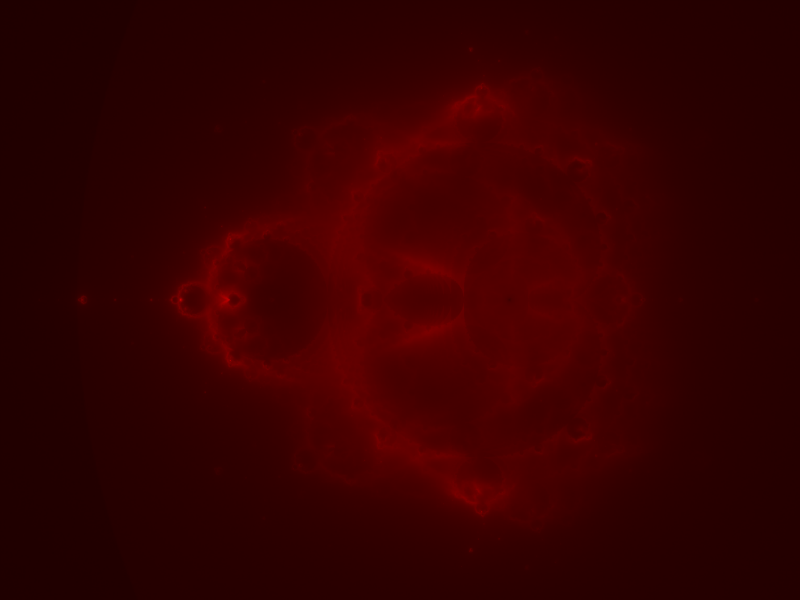## Fractals in Clojure - Distributed Buddhabrot Fractal Using ClojureScript

This one got started because I wanted a large Buddhabrot image on my wall. A large good looking image takes a long time to render, now that we have ClojureScript I thought easiest way to distribute the calculation among machines in the house would be to compile to JavaScript since I've already implemented it in Clojure.

The plan was to compile old code using ClojureScript compiler, fire up a noir instance to collect the data from clients and goto bed, by the time I wake up I thought I would get my giant image. In the end old code did compile to JavaScript I only did minor cosmetic changes and noir did collected the data from clients but clients were way too slow to make any difference.This is a literate program, the code in this document is the executable source, in order to extract it, open this raw file with emacs and run,

M-x org-babel-tangle


It will build the necessary directory structure and export the files into their proper place.

Configuration,

• Plane we want to draw. (left right top bottom)
• Multiply the plane with this number to calculate image size.
• Max iterations.
• Batch size.
• Where to save the data file in case we want to take a break.
(defn config-big []
[[-2.102613 1.200613 -1.237710 1.239710] 600 1000 5000 "fractal.data"])

(defn config-small []
[[-2.102613 1.200613 -1.237710 1.239710] 100 50 1000 "fractal.data"])

(def config config-small)


I am going to skip the math behind the fractal for that you can read my earlier implementation, in a nutshell each clients returns a list of points. For each point we get, we increment a counter in the buffer. In the end we color the fractal based on the number of iterations that passed through that pixel.

Least problematic way to store iteration count turned out to be a 2D array. I started with a map of coordinate to count but kept getting out of memory errors using the default settings. Increasing the memory did not exactly solved the problem cause then serializing that giant map became the problem so I've settled on a integer array. All interactions with the buffer is handled by an agent.

(let [[[left right top bottom] size _ _ data-file] (config)
fractal (agent (make-array Integer/TYPE
(Math/ceil (* size (- bottom top)))
(Math/ceil (* size (- right left)))))]

(defn inc-pixels [coords]
(send fractal (fn [state coords]
(doseq [[x y] coords]
(try
(aset state y x (inc (aget state y x)))
(catch Exception e (println e x y))))
state)
coords))

(defn spit-fractal []
(send fractal
(fn [state]
(doto (java.io.ObjectOutputStream.
(java.io.FileOutputStream. data-file))
(.writeObject state)
(.flush)
(.close))
state)))

(defn slurp-fractal []
(when (.exists (java.io.File. data-file))
(let [in (java.io.ObjectInputStream. (java.io.FileInputStream. data-file))
obj (cast (Class/forName "[[J") (.readObject in))]
(.close in)
(send fractal (fn [_ o] o) obj))))

(defn pixels []
(for [x (range (* size (- right left)))
y (range (* size (- bottom top)))] [x y (aget @fractal y x)])))


Each request to /calculate will fire a Web Worker, each web worker will calculate a vector of valid points once a certain number of points is reached (defined in the configuration) it will make a post request to /receive sending its batch.

(defn log [str]
(js* "console.log(~{str})"))

(let [xhr (.target e)
response (. xhr (getResponseText))]
(log response)))

(defn ^:export init []
(while true
(js/postMessage "Calculating Batch")
(let [batch (calc-batch)]
(js/postMessage "Sending Batch")

(init)

(defpage "/calculate" []
(html
[:html
[:body
[:span {:id "status"}]
[:script {:type "text/javascript"}
"var worker = new Worker('calculate.js');
worker.onmessage = function (event) {
document.getElementById(\"status\").textContent = event.data;
};"]]]))

"OK")


Image is created by iterating over each pixel and color it using sqrt scaling, $$val = 255 * \frac{\sqrt{iterations}}{\sqrt{max-iterations}}$$. This leads to images that are not washed out in the high end of the iteration and also not too pixelated in the low end.

(defn color [iteration max-iterations]
(Color. (int (* 255 (/ (Math/sqrt iteration)
(Math/sqrt max-iterations)))) 0 0))

(let [[[left right top bottom] size _ _ data-file] (config)
width (* size (- right left))
height (* size (- bottom top))]

(defn create-image []
(let [image  (BufferedImage. width height BufferedImage/TYPE_INT_RGB)
graphics (.createGraphics image)
biggest  (apply max (map last (pixels)))]

(doseq [[x y count] (pixels)]
(.setColor graphics (color count biggest))
(.drawLine graphics x y x y))

(javax.imageio.ImageIO/write image "png"
(java.io.File. (str data-file ".png"))))))


In order to create your own fractal after tangling this file compile ClojureScript part,

cljsc source/resources/calculate.cljs '{:optimizations :advanced}' > \
source/resources/public/calculate.js


Start a repl,

lein repl


then start the noir instance,

(server)


finally navigate to http://127.0.0.1:8080 from a bunch of machines. The more you wait the better the picture gets.